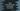# Java program to move all zeroes of an integer array to the start### Java program to move all zeros of an integer array to the start of an array :

In this tutorial, we will learn how to move all zero of an array to the start of it using Java programming language. For example, for the array {1,0,2,0,3,0}, it will become {0,0,0,1,2,3} after the conversion. To solve this problem, we will use the following algorithm :

### Algorithm :

1. The array is given. We will scan the elements from end to first. For example , for the array {1,0,2,0,3,0},we will scan one by one element as 0,3,0,2,0 and 1.
2. Create one variable to store the current index. At first, its value is the last index of the array.
3. Check for each element of the array while scanning, if it is not zero, add the value to the current index of the array.
4. After all values are completed, fill the remaining position of the array with 0.
5. Finally, print out the array.

### Java Program :

``````class Main {
public static void main(String args[]) {
//1
int[] array = {1, 2, 0, 4, 6, 0, 9, 0, 4, 0, 3, 0, 9, 0, 1, 0, 3, 0};

//2
int current = array.length - 1;

//3
for (int i = array.length - 1; i >= 0; i--) {
if (array[i] != 0) {
array[current] = array[i];
current--;
}
}

//4
while (current >= 0) {
array[current] = 0;
current--;
}

//5
for (int i = 0; i < array.length; i++) {
System.out.print(array[i] + " ");
}
}
}``````

### Explanation :

The commented numbers in the above program denote the step number below :

1. array is the given array with all different integer values.
2. Create one integer variable current to store the current index to insert value. At first, its value should be same as the last index of the array.
3. Start one for loop to scan the numbers from end to the start of the array.Check for each element, if it is 0 or not. If not, insert the value to current index and decrement the value of index. Else, continue the loop.
4. After all elements are scanned, fill all remaining position of the array with 0.
5. Finally, print out all elements of the array. It should contain 0 in the beginning.

### Output :

Following is the output of the above program :

``0 0 0 0 0 0 0 0 1 2 4 6 9 4 3 9 1 3 ``